﻿ 噪声环境下复杂流形数据的势能层次聚类算法文章快速检索 高级检索
 重庆邮电大学学报(自然科学版)2018, Vol. 30Issue (6): 848-854  DOI: 10.3979/j.issn.1673-825X.2018.06.0170

### 引用本文YU Xiaofei, GE Hongwei. Hierarchical clustering algorithm based on potentialin complex flow data sets with noise[J]. Journal of Chongqing University of Posts and Telecommunications (Natural Science Edition), 2018, 30(6): 848-854. DOI: 10.3979/j.issn.1673-825X.2018.06.017.### 文章历史

1. 江南大学 轻工过程先进控制教育部重点实验室，江苏 无锡 214122;
2. 江南大学 物联网工程学院，江苏 无锡 214122

Hierarchical clustering algorithm based on potentialin complex flow data sets with noise
YU Xiaofei1,2, GE Hongwei1,21. Key Laboratory of Advanced Process Control for Light Industry, Ministry of Education, Jiangnan University, Wuxi 214122, P. R. China;
2. School of Internet of Things Engineering, Jiangnan University, Wuxi 214122, P. R. China
Foundation Items: The Research Innovation Program for College Graduate of Jiangsu Province(KYLX16_0781);The Research Innovation Program for College Graduate of Jiangsu Province(KYLX16_0782);The Foundation of Priority Academic Program Development of Jiangsu Higher Education Institutions
Abstract: Potential-based hierarchical agglomerative (PHA) clustering uses a new similarity metric to get clustering results more efficiently. Aiming at the problem that PHA can not effectively deal with the complex structure data sets with noise, we proposed a hierarchical clustering algorithm based on potential in complex flow data sets with noise. Firstly, the noise points were identified by means of the potential increase curve; Secondly, the maximum and minimum layers of the data set based on potential were defined, and these two data layers were made to cluster automatically to determine the general framework of the clusters; Finally, clustering of the entire data set was hierarchied based on the precious clustering results. The experiments on artificial data sets show that the new algorithm can deal with the complex flow data sets with noise effectively. The experiments on real data sets show that the new algorithm can get the better clustering results.
Keywords: clustering    potential-based hierarchical agglomerative (PHA)    potential hierarchy    hierarchical agglomerative clustering    noise recognition
0 引言

Yonggang Lu等提出一种基于势能的聚类(clustering by sorting potential values，CSPV)算法，该算法虽提出了基于势能的相似性度量准则，但聚类效果完全依赖于对参数B的选取。针对这一问题，Yonggang Lu等于2013年在《Pattern Recognition》上提出了一种基于势能的快速凝聚层次聚类(potential-based hierarchical agglomerative clustering，PHA)算法。该算法十分便捷高效，首先依据各点的势能和与父节点的距离构造边缘加权树，然后依据树中权值大小使用层次聚类得到最终的聚类结果。PHA算法使用全新的相似性度量准则，并与凝聚层次聚类相结合，在时间上更快速，在精度上可以得到更好的聚类效果。

PHA算法虽然具有上述优点，但该算法只能处理一些结构简单的、簇内势能大小分布明显不同的球形簇，无法处理一些复杂的流形结构簇，而且聚类结果易受到噪声数据的影响。针对这一缺陷，本文提出了噪声环境下复杂流形数据的势能层次聚类算法(hierarchical clustering based on potential in complex flow data with noise，HCPC)。首先，根据势能递增曲线确定势能阈值，找到数据集中的噪声点；其次，在非噪声数据点上，定义出势能最大、最小2层数据，并基于势能的分布特点，提出分层聚类的方法，以确定类簇的大体框架；最后，在分层聚类结果的基础上，对正常点使用层次聚类，同时将噪声点聚到距离自身最近的类簇中去。实验表明，HCPC算法不仅可以识别噪声点，而且能有效地处理复杂的流形数据集，同时在真实数据集上具有更优的聚类效果。

1 PHA算法及其缺陷分析 1.1 PHA算法

PHA算法认为，数据点的势能大小与其概率密度分布是密切相关的。该算法首先根据重力势能的物理意义来计算每个数据的势能。其中，每2点之间的势能Φij定义为

 ${\mathit{\Phi }_{ij}}({r_{ij}}) = \left\{ \begin{array}{l} - \frac{1}{{{r_{ij}}}}, \;\;\;\;\;{\rm{若}}{r_{ij}} \ge \delta \\ - \frac{1}{\delta }, \;\;\;\;\;{\rm{若}}{r_{ij}} < \delta \end{array} \right.$ (1)

(1) 式中：rij是点i与点j之间的欧式距离；δ用来避免分母为零的情况出现。δ的计算方式为

 $Min{D_i} = \mathop {{\rm{min}}}\limits_{{r_{ij}} \ne 0, j = 1, \ldots , N} ({r_{ij}})$ (2)
 $\delta = mean\left( {Min{D_i}} \right)/S$ (3)

(2)—(3)式中：MinDi是点i到其他各点的最短距离；N是数据点的个数；S是一个尺度因子，一般设为10。

 ${\mathit{\Phi }_i} = \sum\limits_{j = 1}^N {{\mathit{\Phi }_{ij}}({r_{ij}})}$ (4)

 ${\hat p_N}(i) = \frac{1}{N}\sum\limits_{j = 1}^N {{\mathit{\Phi }_{ij}}({r_{ij}})}$ (5)

(5) 式中，N表示数据点总数。

 ${\mathit{\Phi }_{ij}} = \left( { - N/\alpha } \right){{\hat p}_N}(i)$ (6)

 $parent\left[ i \right] = \mathop {{\rm{argmin}}}\limits_k ({r_{k, i}}|{\mathit{\Phi }_k} \le {\mathit{\Phi }_i}\;AND\;k \ne i)$ (7)

i到父节点parent[i]的距离ρi

 ${\rho _i} = {r_{i, parent\left[ i \right]}}$ (8)

1.2 PHA算法缺陷分析

PHA算法虽然十分便捷快速，但其只能处理一些簇内势能分布明显不均匀的数据集如图 1所示。然而，针对一些复杂的流形数据集，PHA算法无法得到理想的聚类结果。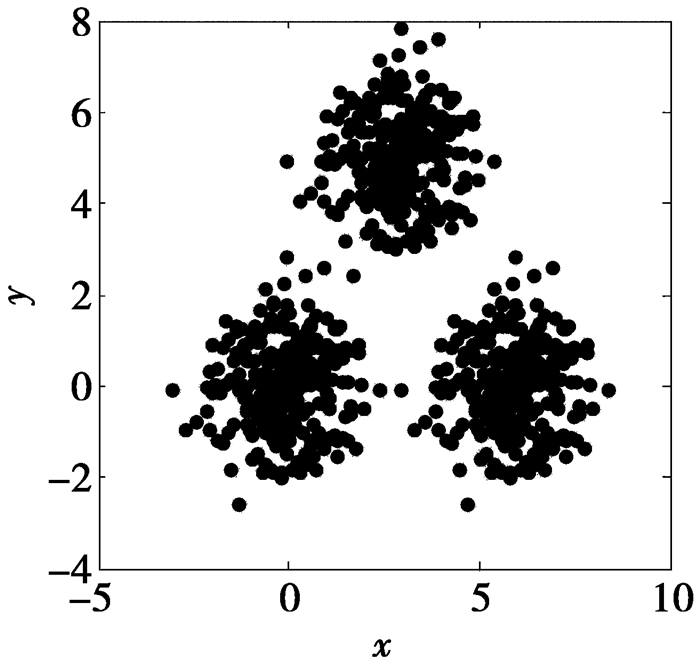图 1 DS1数据集 Figure 1 DS1 data set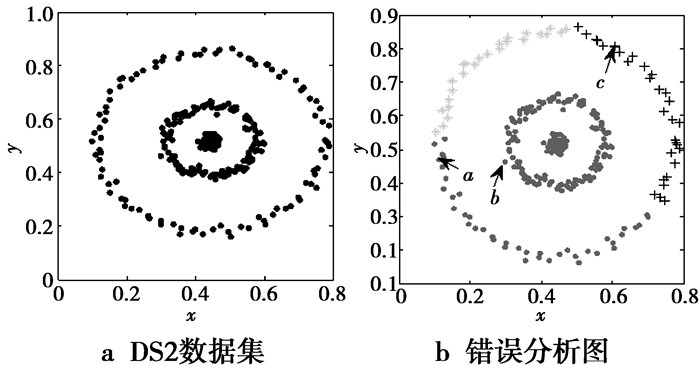图 2 缺陷分析示例 Figure 2 Defect example analysis

2 含噪声的复杂流形数据的势能层次聚类 2.1 识别噪声点

HCPC算法采用构造势能递增曲线的方法识别噪声点。一般情况下，由于各数据集中噪声点的数量远少于正常数据点，且噪声点的分布相对稀疏，因此其势能较大。噪声点判定如图 3所示，在势能递增曲线上，噪声点与正常点之间存在一个拐点，图 3a中的点C即为图 3b中数据集的拐点，拐点之前的点为正常点，拐点之后的点为噪声点，图 3b中的星型点为该数据集被识别的噪声点。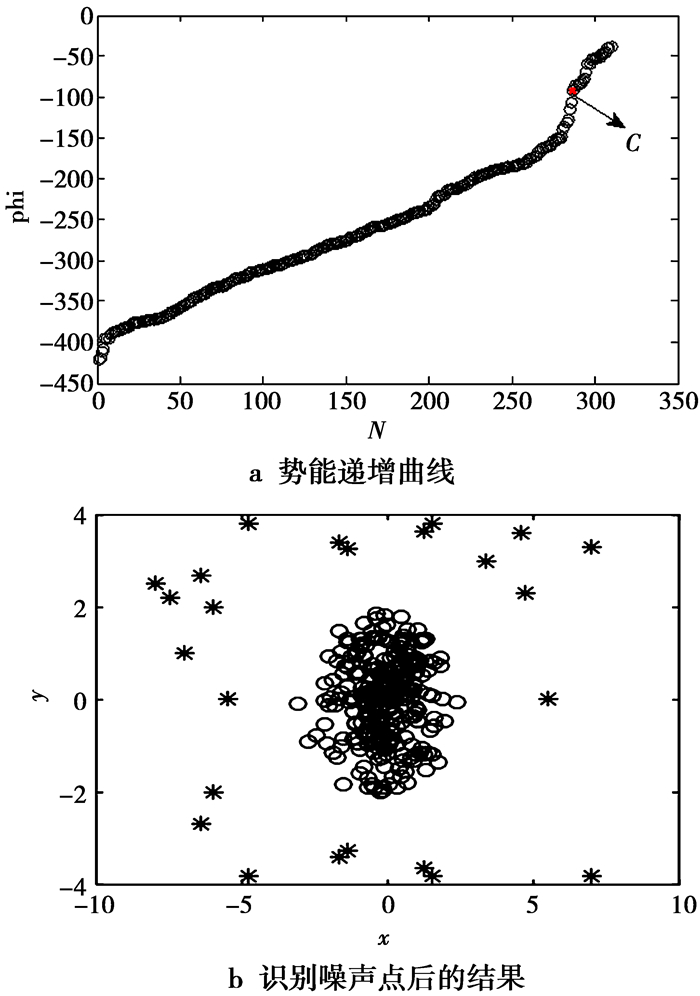图 3 噪声点判定 Figure 3 Determination of noise points
2.2 势能的分层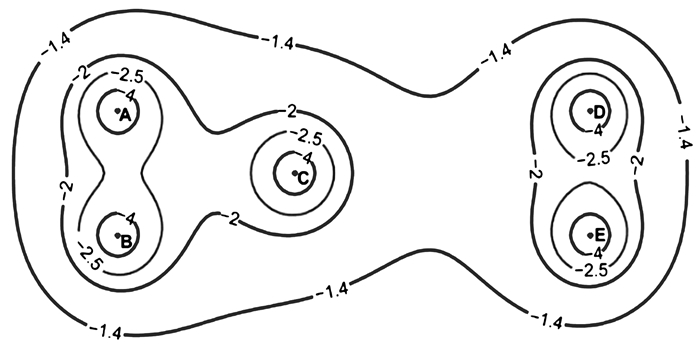图 4 势能分布图 Figure 4 Graph ofpotential field

 ${S_{\max }} = \left\{ {i|{\mathit{\Phi }_i} \ge {\mathit{\Phi }_n}} \right\}$ (9)

 ${S_{\min }} = \left\{ {i|{\mathit{\Phi }_i} \le {\mathit{\Phi }_p}} \right\}$ (10)

(9)—(10)式中：Φn为去除噪声点后，势能从大到小排序后，第$\left\lfloor {N' \times 0.25} \right\rfloor$个数据Φp为势能从小到大排序后，第$\left\lfloor {N' \times 0.25} \right\rfloor$个数据

 $N' = N - n$ (11)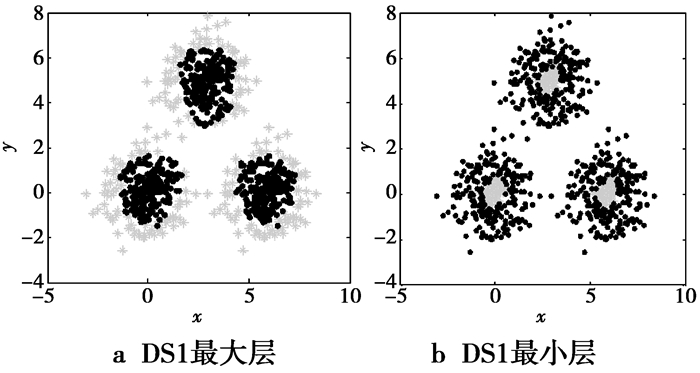图 5 DS1分层 Figure 5 Layer of DS1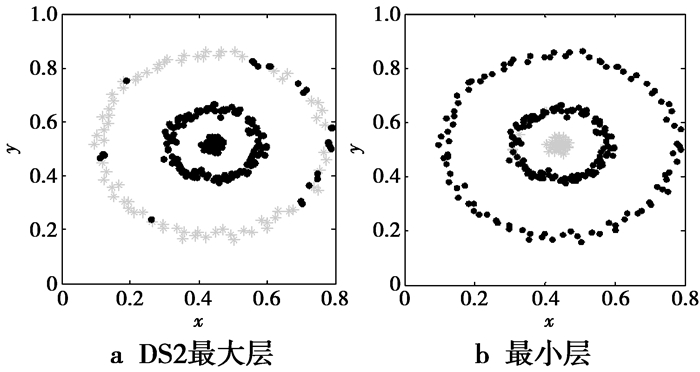图 6 DS2分层 Figure 6 Layer of DS2

 ${\gamma _i} = |{\mathit{\Phi }_i}|\cdot{\rho _i} = - {\mathit{\Phi }_i}\cdot\rho$ (12)

2层数据的聚类结果如图 7所示，图 7中黑色圆点为未聚类数据点。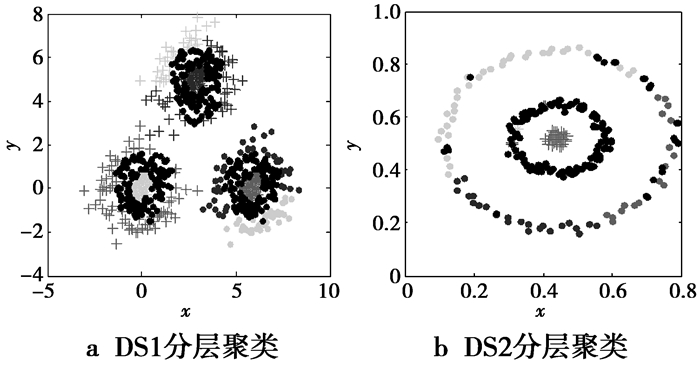图 7 分层聚类结果 Figure 7 Clustering result of hierarchy clustering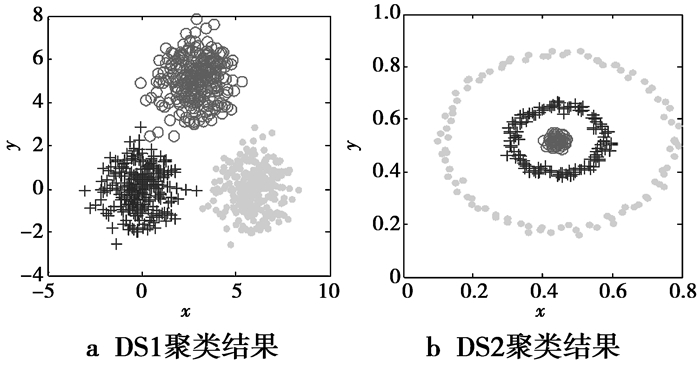图 8 DS1与DS2聚类结果 Figure 8 Clustering results of DS1 and DS2

2.3 HCPC算法步骤

HCPC算法流程简单描述如下。

2.4 算法复杂度分析

HCPC算法的时间复杂度主要由3部分组成：计算势能和距离；在最大、最小层数据上对γi值分类；使用最小类间距离进行层次聚类。假设数据点个数为n，则计算势能和距离的时间复杂度为O(n2)，在最大、最小2层数据上对γi值分类的时间复杂度为O(n)，最后层次聚类的时间复杂度为O(n2)，因此，HCPC算法的时间复杂度为O(n2)。文献中介绍PHA算法的时间复杂度为O(n2)，可见，HCPC算法在没有增加算法时间复杂度的同时，提高了算法的聚类效果。

3 实验分析

3.1 人工数据集的实验结果分析表 1 4个人工数据集信息 Table 1 Four synthetic data sets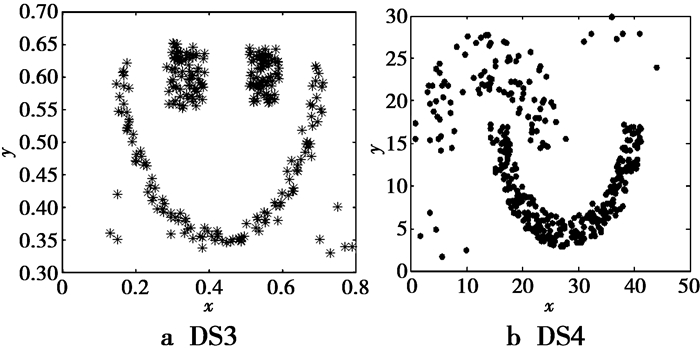图 9 DS3，DS4数据集 Figure 9 DS1 and DS2 data sets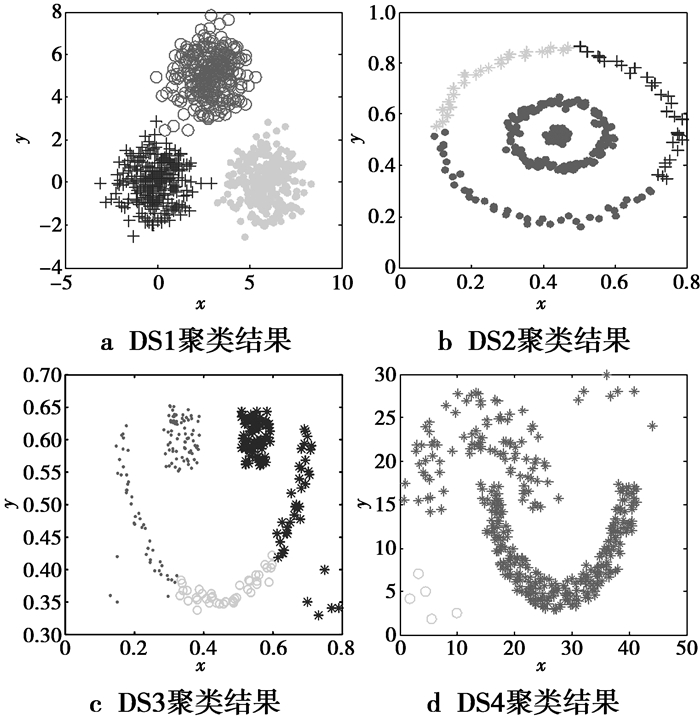图 10 PHA在人工数据集的聚类结果 Figure 10 Clustering results onsynthetic datasets by PHA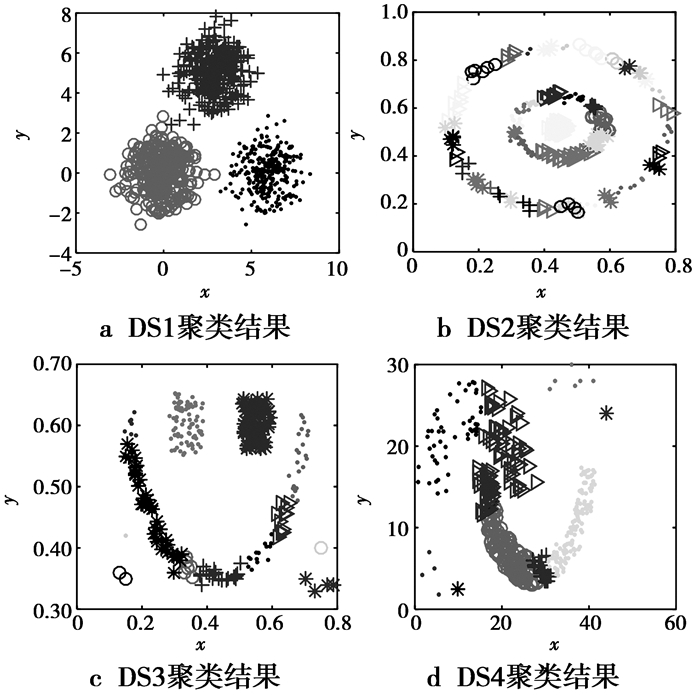图 11 CSPV在人工数据集的聚类结果 Figure 11 Clustering results on synthetic datasets by CSPV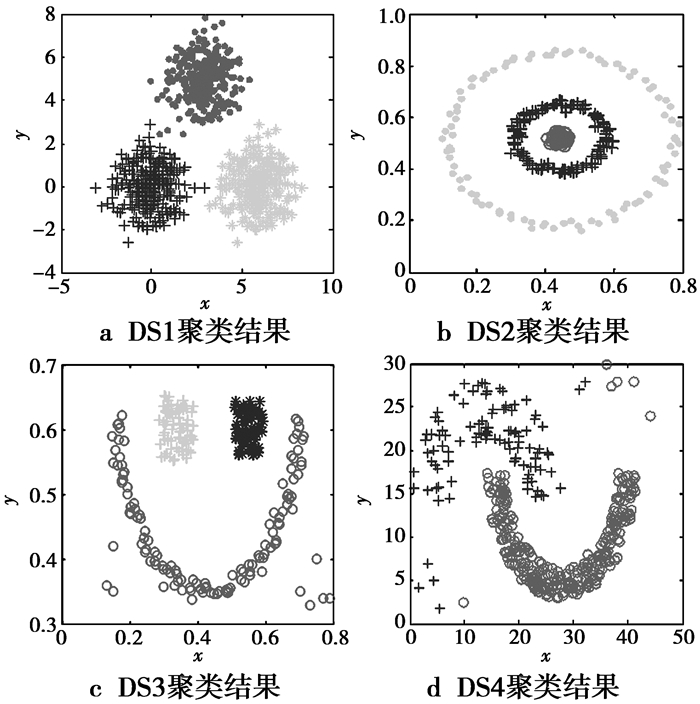图 12 HCPC在人工数据集的聚类结果 Figure 12 Clustering results on synthetic datasets by HCPC

3.2 真实数据集的实验结果分析表 2 5个真实数据集信息 Table 2 Five real data sets表 3 PHA算法在真实数据集上的聚类指标值 Table 3 Index values of PHA on real data sets表 4 HCPC算法在真实数据集上的聚类指标值 Table 4 Index values of HCPC on real data sets表 5 CSPV算法在真实数据集上的聚类指标值 Table 5 Index values of CSPV on real data sets

4 结束语

  WILLIAMSON B, GUYON I. Clustering: science or art?[J]. Mach Learn Res, 2012, 27(2): 65-80.  张勇, 王庆, 夏长红, 等. 离散多目标量子微粒群聚类算法[J]. 模式识别与人工智能, 2017, 30(3): 204-213. ZHANG Yong, WANG Qing, XIA Changhong, et al. Discrete Multi-objective Quantum Particle Swarm Optimization Clustering Algorithm[J]. Pattern Recognition and Artificial Intelligence, 2017, 30(3): 204-213.  朱杰, 陈黎飞. 核密度估计的聚类算法[J]. 模式识别与人工智能, 2017, 30(5): 439-447. ZHU Jie, CHEN Lifei. Clustering Algorithm with Kernel Density Estimation[J]. Pattern Recognition and Artificial Intelligence, 2017, 30(5): 439-447.  JAINA K. Data clustering: 50 years beyond K-means[J]. Pattern Recognition, 2010, 31(8): 651-666. DOI:10.1016/j.patrec.2009.09.011  VELMURUGAN T. Performance based analysis between k-Means and Fuzzy C-Means clustering algorithms for connection oriented telecommunication data[J]. APPLIED SOFT COMPUTING, 2014, 19(46): 134-146.  PARK H S, JUN C H. A simple and fast algorithm for K-medoids clustering[J]. Expert Systems with Applications, 2009, 36(2): 3336-3341.  GUHAS. Cure: an efficient clustering algorithm for large databases[C]//Proceeding of the ACM SIGMOD International Conference on Management of Data.New York: Information Systems, 1998: 73-84.  WILCOX H, NICHOL R C, ZHAO G B. Simulation tests of galaxy cluster constraints on chameleon gravity[J]. Monthly Notices of the Royal Astronomical Society, 2016, 452(2): 715-725.  VISWANATH P, BABU S V. Rough-DBSCAN:A fast hybrid density based clustering method for large data sets[J]. Pattern Recognition Letters, 2009, 30(16): 1477-1488. DOI:10.1016/j.patrec.2009.08.008  KALITA H K. A New Algorithm for Ordering of Points to Identify Clustering Structure Based on Perimeter of Triangle: OPTICS(BOPT)[C]//ladcom. IEEE Computer Society.New York: IEEE Press, 2007: 523-528.  ANSARI S, CHETLUR S, PRABHU S, et al. An Overview of Clustering Analysis Techniques used in Data Miniing[J]. International Journal of Emerging Technology and Advanced Engineering, 2013, 3(12): 284-286.  AMINI A, WAH T Y, SAYBANI M R, et al. A study of density-grid based clustering algorithms on data streams[C]//Fuzzy Systems and Knowledge Discovery (FSKD), 2011 Eighth International Conference on.New York: IEEE Press, 2011, 3: 1652-1656.  YONGGANG Lu, YI Wan. Clustering by Sorting Potential Values(CSPV):A novel potential-based clustering method[J]. Pattern Recognition, 2012, 45(9): 3512-3522. DOI:10.1016/j.patcog.2012.02.035  YONGGANG Lu, YI Wan. PHA: A fast potential-based hierarchical agglomerative clustering method[J]. Pattern Recognition, 2013, 46(5): 1227-1239. DOI:10.1016/j.patcog.2012.11.017  PARZEN E. On estimation of a probability density function and mode[J]. Annals of Mathematical Statistic, 1962, 33(07): 1065-1076.  LI Caiyun, WAN Yi. Research on Improved Hierarchical Clustering Algorithm Based on Density[D]. Lanzhou: Lanzhou University, 2016.  于晓飞, 葛洪伟. 自动确定聚类中心的势能聚类算法[J]. 计算机科学与探索, 2017, 12(6): 1004-1012. YU Xiaofei, GE Hongwei. Potential clustering by Automatic Determination of Cluster Center[J]. Journal of Frontiers of Computer Science and Technology, 2017, 12(6): 1004-1012.  LICHMAN M.UCI machine learning repository[EB/OL].(2013-12-23)[2017-10-10].http://archive.ics.uci.edu/ml.  FOWLKES E B. A method for comparing two hierarchical clusterings[J]. Journal of the American Statistical Association, 1983, 78(383): 553-569. DOI:10.1080/01621459.1983.10478008  YANG Y, JIN F, MOHAMED K. Survey of clustering validity evaluation[J]. Application Research of Computers, 2008, 25(6): 1630-1632.  VINH N X, EPPS J, BAILEYJ.Information theoretic measures for clusterings comparison: is a correction for chance necessary?[C]//Proceeding ICML'09. Proceedings of the 26th Annual International Conference on Machine Learning. Montreal, Quebec, Canada: ACM, 2009: 1073-1080.